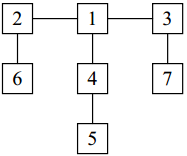## Balancing Act

Consider a tree T with N (1 ≤ N ≤ 20,000) nodes numbered 1 … N. Deleting any node from the tree yields a forest: a collection of one or more trees. Define the balance of a node to be the size of the largest tree in the forest T created by deleting that node from T. For example, consider the tree:Deleting node 4 yields two trees whose member nodes are {5} and {1, 2, 3, 6, 7}. The larger of these two trees has five nodes, thus the balance of node 4 is five. Deleting node 1 yields a forest of three trees of equal size: {2, 6}, {3, 7}, and {4, 5}. Each of these trees has two nodes, so the balance of node 1 is two.

For an input tree, calculate the node that has the minimum balance. If multiple nodes have equal balance, output the one with the lowest number.

### Input Format

The first line of input contains a single integer N. The next N−1 lines contain two space-separated node numbers that are the endpoints of an edge in the tree. No edge will be listed twice, and all edges will be listed.

### Output Format

The output should consist of one line with two space-separated integers — the number of the node with minimum balance, and the balance of that node.

```7
2 6
1 2
1 4
4 5
3 7
3 1
```

### Sample Output

```1 2
```

Point Value: 15
Time Limit: 2.00s
Memory Limit: 16M